Courses

# Test: Thin & Thick Cylinders And Spheres - 1

## 10 Questions MCQ Test Topicwise Question Bank for GATE Civil Engineering | Test: Thin & Thick Cylinders And Spheres - 1

Description
This mock test of Test: Thin & Thick Cylinders And Spheres - 1 for Civil Engineering (CE) helps you for every Civil Engineering (CE) entrance exam. This contains 10 Multiple Choice Questions for Civil Engineering (CE) Test: Thin & Thick Cylinders And Spheres - 1 (mcq) to study with solutions a complete question bank. The solved questions answers in this Test: Thin & Thick Cylinders And Spheres - 1 quiz give you a good mix of easy questions and tough questions. Civil Engineering (CE) students definitely take this Test: Thin & Thick Cylinders And Spheres - 1 exercise for a better result in the exam. You can find other Test: Thin & Thick Cylinders And Spheres - 1 extra questions, long questions & short questions for Civil Engineering (CE) on EduRev as well by searching above.
QUESTION: 1

### A thick cylinder is subjected to an internal pressure of 60 MPa. If the hoop stress on the outer surface is 150 MPa, then the hoop stress on the internal surface is

Solution: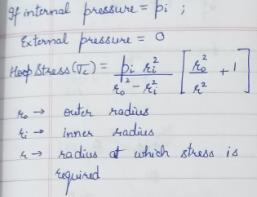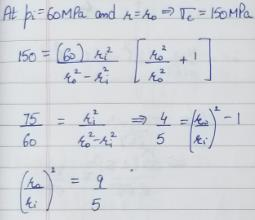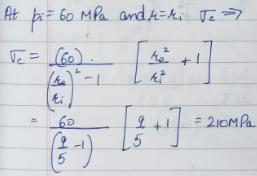QUESTION: 2

Solution:
QUESTION: 3

### From design point of view, spherical pressure vessels are preferred over cylindrical pressure vessels because they

Solution:
QUESTION: 4

A thin cylindrical shell of internal diameter D and thickness 't' is subjected to internal pressure ‘p’. The change in diameter is given by

Solution: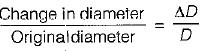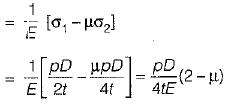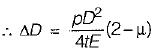QUESTION: 5

A thin cylinder of thickness ‘t’, width ‘b’ and internal radius ‘r’ is subjected to a pressure ‘p’ on the entire internal surface. What is the change in radius of the cylinder? (μ is the Poisson’s ratio and E is the modulus of elasticity)?

Solution: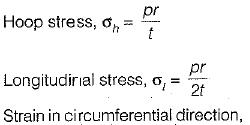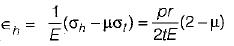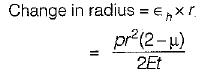QUESTION: 6

Auto-frettage is the method of

Solution:
QUESTION: 7

Circumferential stress in a cylindrical steel boiler shell under internal pressure is 80 MPa. Young’s modulus of elasticity and Poisson's ratio are respectively 2 x 105 MPa and 0.28. The magnitude of circumferential strain in the boiler shell will be

Solution: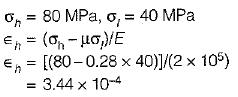QUESTION: 8

Hoop stress and longitudinal stress in a boiler shell under internal pressure are 100 MN/m2 and 50 MN/m2respectively. Young’s modulus of elasticity and Poisson’s ratio of the shell material are 200 GN/m2 and 0.3 respectively. The hoop strain in boiler shell is

Solution: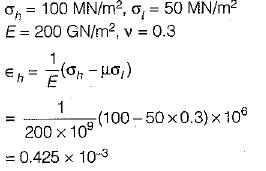QUESTION: 9

A steel hub of 100 mm internal diameter and uniform thickness of 10 mm was heated to a temperature of 300°C to shrink-fit it on a shaft. On cooling a crack developed parallel to the direction of the length of the hub. Consider the following factors in this regard:
1. Tensile hoop stress
2. Tensile radial stress
3. Compressive hoop stress
4. Compressive radial stress

The cause of failure is attributed to

Solution:

A crack in the hub shall develop parallel to the direction of its length due to tensile hoop stress.

QUESTION: 10

A thin cylinder of unit length, thickness ‘t’ and radius ‘r’ is subjected to internal pressure ‘p’. What is the circumferential stress?

Solution:

Circumferential stress is nothing but hoop stress.